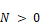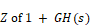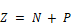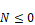#### Nyquist stability criterion

The closed-loop system, whose open-loop transfer function is GH(s) is stable if and only if N = -P ≤ 0 , where:

P ≡ number of poles of GH(s) in the right-half plane (P ≥ 0)

N ≡  total number of clockwise encirclements of the (-1,0) point (GH(s) = -1) () in the GH(s) plane

Ifthe number of zerosin the right-half plane is determined byIfthe (-1,0) point is not enclosed by the Nyquist stability plot. Converselyif the region to the right of the contour in the clockwise direction does not include the (-1,0) point.